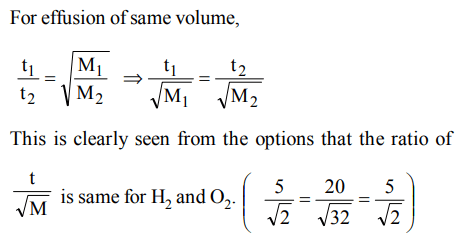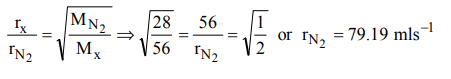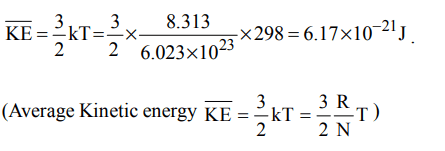## States of Matter Questions and Answers Part-2

1. The densities of two gasses are in the ratio of 1: 16. The ratio of their rates of diffusion is
a) 16:1
b) 4:1
c) 1:4
d) 1:16

Explanation: $\frac{r_{1}}{r_{2}}= \sqrt{\frac{d_{2}}{d_{1}}} = \sqrt{\frac{16}{1}} = 4:1$

2. At what temperature, the rate of effusion of $N_{2}$ would be 1.625 times than that of $SO_{2}$ at 50o C ?
a) 110 K
b) 173 K
c) 373 K
d) 273 K

Explanation:3. The rate of diffusion of methane at a given temperature is twice that of X. The molecular weight of X is
a) 64.0
b) 32.0
c) 40.0
d) 80.0

Explanation: $\frac{2}{1}= \sqrt{\frac{M_{x}}{16}}$
Mx = 64

4. X ml of $H_{2}$ gas effuse through a hole in a container in 5 seconds. The time taken for the effusion of the same volme of the gas specified below under identical conditions is
a) 10 seconds : He
b) 20 seconds :$O_{2}$
c) 25 seconds : CO
d) 55 seconds :$CO_{2}$

Explanation:5. The rate of diffusion of a gas having molecular weight just double of nitrogen gas is $56mls^{-1}$ . The rate of diffusion of nitrogen will be
a) $79.19mls^{-1}$
b) $12.0mls^{-1}$
c) $56.0.0mls^{-1}$
d) $90.0mls^{-1}$

Explanation:6. Which pair of the gaseous species diffuse through a small jet with the same rate of diffusion at same P and T
a) NO, CO
b) $NO,CO_{2}$
c) $NH_{3},PH_{3}$
d) $NO,C_{2}H_{6}$

Explanation: NO and C2H6 both have same molecular weight. Hence their rate of diffusion will be same

7. The rate of diffusion of $SO_{2},CO_{2},PCI_{3} and SO_{3}$     are in the following order
a) $PCI_{3}> SO_{3}>SO_{2}>CO_{2}$
b) $CO_{2}>SO_{2}>PCI_{3}> SO_{3}$
c) $SO_{2}> SO_{3}>PCI_{3}>CO_{2}$
d) $CO_{2}>SO_{2}> SO_{3}>PCI_{3}$

Explanation: $Rate \propto \sqrt{\frac{1}{M}}$    The smaller the value of M the more is the rate of diffusion

8. Which of the following expression correctly represents the relationship between the average molar kinetic energy $\overline{K}E$  of CO and $N_{2}$ molecules at the same temperature
a) $\overline{K}E_{CO}=\overline{K}E_{N_{2}}$
b) $\overline{K}E_{CO}>\overline{K}E_{N_{2}}$
c) $\overline{K}E_{CO}<\overline{K}E_{N_{2}}$
d) Can not be predicted unless the volumes of the gases are not given

Explanation: At the same temperature KE is the same, as KE  $\propto$  T

9. Kinetic theory of gases presumes that the collisions between the molecules to be perfectly elastic because
a) the gas molecules are tiny particles and not rigid in nature
b) the temperature remains constant irrespective of collision
c) collision will not split the molecules
d) the molecules are large particleand rigid in nature

a) $6.17 × 10^{-21}kJ$
b) $6.17 × 10^{-21}J$
c) $6.17 × 10^{-20}J$
d) $7.16 × 10^{-20}J$